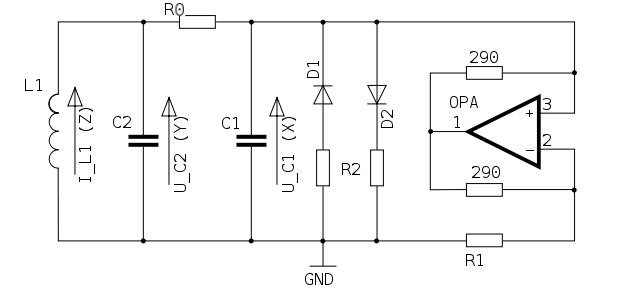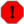## chuap

chuap — Simulates Chua's oscillator, an LRC oscillator with an active resistor, proved capable of bifurcation and chaotic attractors, with k-rate control of circuit elements.

## Description

Plugin opcode in chua. This opcode is part of the plugin repository and has to be installed separately. The plugin repository can be found here: https://github.com/csound/plugins

Simulates Chua's oscillator, an LRC oscillator with an active resistor, proved capable of bifurcation and chaotic attractors, with k-rate control of circuit elements.

## Syntax

`aI3, aV2, aV1 chuap kL, kR0, kC2, kG, kGa, kGb, kE, kC1, iI3, iV2, iV1, ktime_step`

## Initialization

iI3 -- Initial current at G

iV2 -- Initial voltage at C2

iV1 -- Initial voltage at C1

## Performance

kL -- Inductor L (L1 in the diagram)

kR0 -- Resistor R0 (R0 in the diagram)

kC1 -- Capacitor C1

kC2 -- Capacitor C2

kG -- Resistor G (part of the active resistor, R1 in the diagram). The G parameter is the time step, which is needed to get the same slope of the piecewise discontinuity from Ga and Gb for all sampling rates.

kGa -- Resistor V (nonlinearity term of the active resistor, one of the R2's in the diagram)

kGb -- Resistor V (nonlinearity term of the active resistor, one of the R2's in the diagram)

kE -- Size of the piecewise discontinuity simulating the active resistor

ktime_step -- Delta time in the difference equation, can be used to more or less control pitch.

Chua's oscillator is a simple LRC oscillator with an active resistor. The oscillator can be driven into period bifurcation, and thus to chaos, because of the nonlinear response of the active resistor.Diagram of Chua's Oscillator Circuit

The circuit is described by a set of three ordinary differential equations called Chua's equations:

dI3      R0      1
--- =  - -- I3 - - V2
dt       L       L

dV2    1       G
--- = -- I3 - -- (V2 - V1)
dt    C2      C2

dV1    G              1
--- = -- (V2 - V1) - -- f(V1)
dt    C1             C1

where f() is a piecewise discontinuity simulating the active resistor:

f(V1) = Gb V1 + - (Ga - Gb)(|V1 + E| - |V1 - E|)

A solution of these equations (I3,V2,V1)(t) starting from an initial state (I3,V2,V1)(0) is called a trajectory of Chua's oscillator. The Csound implementation is a difference equation simulation of Chua's oscillator with Runge-Kutta integration.

NoteThis algorithm uses internal non linear feedback loops which causes audio result to depend on the orchestra sampling rate. For example, if you develop a project with sr=48000Hz and if you want to produce an audio CD from it, you should record a file with sr=48000Hz and then downsample the file to 44100Hz using the src_conv utility.
WarningBe careful! Some sets of parameters will produce amplitude spikes or positive feedback that could damage your speakers.

## Examples

Here is an example of the chuap opcode. It uses the file chuap.csd.

Example 140. Example of the chuap opcode.

```<CsoundSynthesizer>
<CsOptions>
; Select audio/midi flags here according to platform
-odac     ;;;RT audio out
; For Non-realtime ouput leave only the line below:
; -o chuap.wav -W ;;; for file output any platform
</CsOptions>
<CsInstruments>

sr     = 44100
ksmps  = 32
nchnls = 2
0dbfs  = 1

gibuzztable ftgen 1, 0, 16384, 10, 1

instr 1

istep_size    =       p4
iL            =       p5
iR0           =       p6
iC2           =       p7
iG            =       p8
iGa           =       p9
iGb           =       p10
iE            =       p11
iC1           =       p12
iI3           =       p13
iV2           =       p14
iV1           =       p15

iattack       =       0.02
isustain      =       p3
irelease      =       0.02
p3            =       iattack + isustain + irelease
iscale        =       1.0
adamping      linseg  0.0, iattack, iscale, isustain, iscale, irelease, 0.0
aguide        buzz    0.5, 440, sr/440, gibuzztable
aI3, aV2, aV1 chuap   iL, iR0, iC2, iG, iGa, iGb, iE, iC1, iI3, iV2, iV1, istep_size
asignal       balance aV2, aguide

endin

</CsInstruments>
<CsScore>
;        Adapted from ABC++ MATLAB example data.
//             time_step      kL           kR0         kC2              kG            kGa           kGb          kE          kC1           iI3                     iV2                     iV1
; torus attractor ( gallery of attractors )
i 1 0 20       .1            -0.00707925   0.00001647  100              1             -.99955324    -1.00028375  1          -.00222159     -2.36201596260071       3.08917625807226e-03    3.87075614929199
; heteroclinic orbit
i 1 + 20       .425           1.3506168    0           -4.50746268737  -1             2.4924        .93          1           1             -22.28662665            .009506608              -22.2861576
; periodic attractor (torus breakdown route)
i 1 + 20       .05            0.00667      0.000651    10              -1             .856          1.1          1           .06           -20.200590133667        .172539323568344        -4.07686233520508
; torus attractor (torus breakdown route)'
i 1 + 20       0.05           0.00667      0.000651    10              -1             0.856         1.1          1           0.1            21.12496758             0.03001749              0.515828669

</CsScore>
</CsoundSynthesizer>
```

## Credits

 Inventor of Chua's oscillator: Leon O. Chua Author of MATLAB simulation: James Patrick McEvoy MATLAB Adventures in Bifurcations and Chaos (ABC++) Author of Csound port: Michael Gogins

New in Csound version 5.09

Note added by François Pinot, August 2009